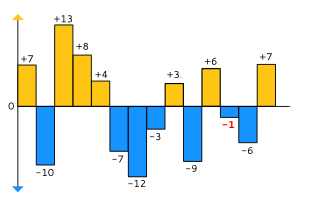# Temperatures

Codingame: Temperatures
Original Problem

##### The Goal

In this exercise, you have to analyze records of temperature to find the closest to zero.Sample temperatures
Here, -1 is the closest to 0.

##### Rules

Write a program that prints the temperature closest to 0 among input data. If two numbers are equally close to zero, positive integer has to be considered closest to zero (for instance, if the temperatures are -5 and 5, then display 5).

##### Game Input

Your program must read the data from the standard input and write the result on the standard output.
Input
Line 1: N, the number of temperatures to analyze
Line 2: A string with the N temperatures expressed as integers ranging from -273 to 5526
Output
Display 0 (zero) if no temperatures are provided. Otherwise, display the temperature closest to 0.
Constraints
0 ≤ N < 10000

##### Java Solution
```class Solution {

public static void main(String args[]) {
Scanner in = new Scanner(System.in);
int n = in.nextInt(); // the number of temperatures to analyse
if (in.hasNextLine()) {
in.nextLine();
}
String temps = in.nextLine(); // the n temperatures expressed as integers ranging from -273 to 5526
int minForPositives = 5526;
int maxForNegatives = -273;
int result = 0;
String[] tempsStr = new String[n];
tempsStr = temps.split(" ");
int[] numbers = new int[n];

for (int i = 0; i < n; i++) {
numbers[i] = Integer.parseInt(tempsStr[i]);
}

for (int i = 0; i < n; i++) {
if (numbers[i] < 0 && maxForNegatives < numbers[i]) maxForNegatives = numbers[i];
if (numbers[i] > 0 && minForPositives > numbers[i]) minForPositives = numbers[i];
}

if (Math.abs(maxForNegatives) >= minForPositives) {
result = minForPositives;
}
if (Math.abs(maxForNegatives) < minForPositives) {
result = maxForNegatives;
}
if (n == 0) {
result = 0;
}
if (n == 1 && numbers == 5526) {
result = 5526;
}
System.out.println(result);
}
}```